# Why Use This?

`for (int i = 0; i < n; i++) { // O(n)    for (int j = 0; j < n; j++) { // O(n), makes this whole loop O(n^2)    }}for (int i = 0; i < n; i++) { // O(n)}int i = 1; // O(1)i += 1; // O(1)i += 1; // O(1)return i; // O(1)`

# Big-O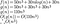We prove f(n) is O(n³) by creating a function that eventually will be greater than or equal to every output of f(n). Note how at the end we only care about n³ and not 110. This is because given a large enough input, the 110 becomes irrelevant to how our function grows compared to the n³.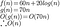We don’t always have to keep each term. Here we were able to drop the second term. Whatever terms we choose for g(n) is arbitrary, so long as it fulfills what we need.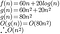Notice how in here we proved f(n) is O(n²), not O(n).

# Big-Theta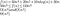Since f(n) is shown to be bounded above and below by n³, we are able to show how on average, it will be n³.

# Further Examples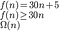Since both the Big-O and Big-Omega are the same, we can say f(n) is Θ(n)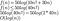Remember some algebra rules. While we could say f(n) is O(log(n²)) (log(n²) is the same as 2log(n)), we could simplify further.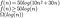Since f(n) is O(log(n)) and Ω(log(n)), we can also say f(n) is Θ(log(n))

Computer Science Major at the University of Central Florida.

## More from Jose Iciano

Computer Science Major at the University of Central Florida.